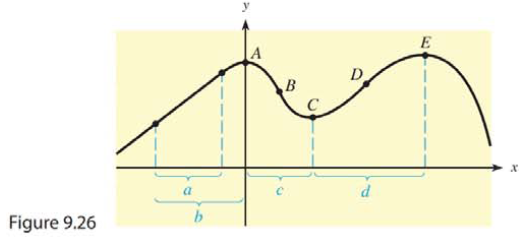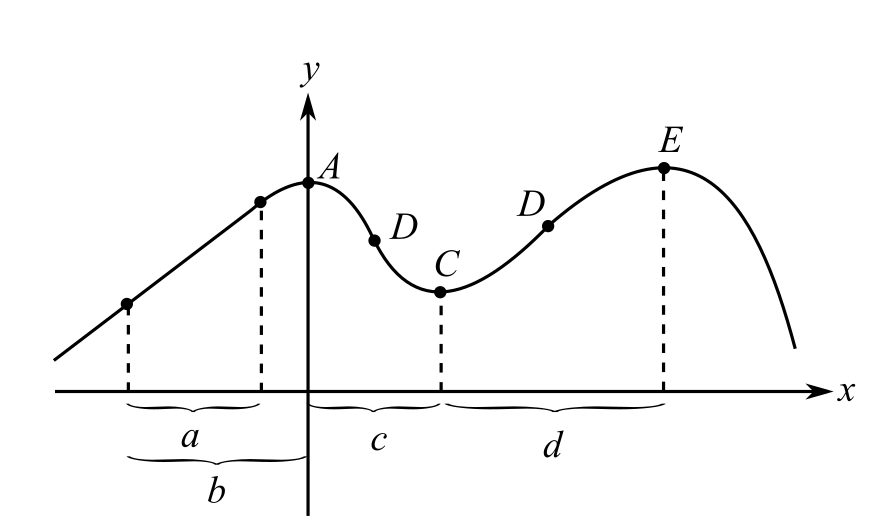Chapter 9.3, Problem 32EMathematical Applications for the ...

12th Edition
Ronald J. Harshbarger + 1 other
ISBN: 9781337625340

Solutions

Chapter
SectionMathematical Applications for the ...

12th Edition
Ronald J. Harshbarger + 1 other
ISBN: 9781337625340
Textbook Problem

Because the derivative of a function represents both the slope of the tangent to the curve and the instantaneous rate of change of the function, it is possible to use information about one to gain information about the other. In Problems 31 and 32, use the graph of the function y = f ( x ) given in Figure 9.26.(a) At what point(s) A through E does the rate of change of f(x) change from positive to negative? (b) At what point(s) A through E does the rate of change of f(x) change from negative to positive?

(a)

To determine

The points at which the rate of change is from positive to negative from the figure. Instantaneous value and slope both are basically the derivatives of the function.Explanation

Given Information:

Instantaneous value and slope both are basically the derivatives of the function. The graph is given as

Explanation:

Consider the provided statement,

Instantaneous value and slope both are basically the derivatives of the function. The graph is given as

Slope is given by the formula

yy1=m(xx1)

Where m is the slope of the line

(b)

To determine

The points at which the rate of change is from negative to positive from the figure. Instantaneous value and slope both are basically the derivatives of the function.Still sussing out bartleby?

Check out a sample textbook solution.

See a sample solution

The Solution to Your Study Problems

Bartleby provides explanations to thousands of textbook problems written by our experts, many with advanced degrees!

Get Started

Evaluate the integral. 4.ye0.2ydy

Calculus: Early Transcendentals

Evaluate the integral. 01(u+2)(u3)du

Single Variable Calculus: Early Transcendentals, Volume I

Given: AEBDEC AEDE Prove: AEBDEC

Elementary Geometry For College Students, 7e

Rectangular coordinates of the point with polar coordinates are: (−1, 0) (0, 1) (0, −1) (1, 0)

Study Guide for Stewart's Single Variable Calculus: Early Transcendentals, 8th